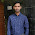## Monday, September 10, 2012

### Electrical Machines: Part 6

 In a transformer the voltage regulation will be zero when it operates at
(a) Unity p.f.
(b) Leading p.f.
(c) Lagging p.f.
(d) Zero p.f. leading.

Answer: B
 In a stepper motor the angular displacement
(a) Can be precisely controlled.
(b) It cannot be readily interfaced with micro computer based controller.
(c) The angular displacement cannot be precisely controlled.
(d) It cannot be used for positioning of work tables and tools in NC machines.

Answer: A
 The power factor of a squirrel cage induction motor is
(a) Low at light load only.
(b) Low at heavy load only.
(c) Low at light and heavy load both.
(d) Low at rated load only.

Answer: A
 The generation voltage in India is usually
(a) Between 11 KV and 33 KV.
(b) Between 132 KV and 400 KV.
(c) Between 400 KV and 700 KV.
(d) None of the above.

Answer: A
 When a synchronous motor is running at synchronous speed, the damper winding produces
(a) Damping torque.
(b) Eddy current torque.
(c) Torque aiding the developed torque.
(d) No torque.

Answer: D
 If a transformer primary is energised from a square wave voltage source, its output voltage will be
(a) A square wave.
(b) A sine wave.
(c) A triangular wave.
(d) A pulse wave.

Answer: A
 A salient pole synchronous motor is running at no load. Its field current is switched off. The motor will
(a) Come to stop.
(b) Continue to run at synchronous speed.
(c) Continue to run at a speed slightly more than the synchronous speed.
(d) Continue to run at a speed slightly less than the synchronous speed.

Answer: B
 The frequency of the rotor current in a 3 phase 50 Hz, 4 pole induction motor at full load speed is about
(a) 50 Hz.
(b) 20 Hz.
(c) 2 Hz.
(d) Zero.

Answer: C
 The speed of a dc motor can be controlled by varying
(a) Its flux per pole
(b) Resistance of armature circuit
(c) Applied voltage
(d) All of the above

Answer: D
 Regarding Ward-Leonard system of speed control which statement is false?
(a) It is usually used where wide and very sensitive speed control is required
(b) It is used for motors having ratings from 750kW to 4000kW
(c) Capital outlay involved in the system is right since it uses two extra machines
(d) It gives a speed range of 10:1 but in one direction only
(e) It has low overall efficiency especially at light loads

Answer: D
9:48 AM

#### 1 comment:

1.Question No 6 the answer is PULSE WAVE